# 指数定律一个数的指数代表把多少个这个数 乘在一起。 在这例子：82 = 8 × 8 = 64 用文字来说：82 也可以叫 "8的2次方"、"8的2次幂" 或 "8的平方"

### 例子：a7

a7 = a × a × a × a × a × a × a = aaaaaaa

## 所有你需要知道的……

"指数定律"基于三个理念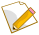指数代表在乘法里用多少次那个数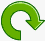负指数的意思是，因为乘的相反是除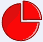分数指数，像 1/n 的意思是取n次方根：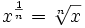## 指数定律

x1 = x 61 = 6
x0 = 1 70 = 1
x-1 = 1/x 4-1 = 1/4
xmxn = xm+n x2x3 = x2+3 = x5
xm/xn = xm-n x6/x2 = x6-2 = x4
(xm)n = xmn (x2)3 = x2×3 = x6
(xy)n = xnyn (xy)3 = x3y3
(x/y)n = xn/yn (x/y)2 = x2 / y2
x-n = 1/xn x-3 = 1/x3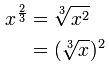## 定律的解释

。。。等等。。。52 1 × 5 × 5 25
51 1 × 5 5
50 1 1
5-1 1 ÷ 5 0.2
5-2 1 ÷ 5 ÷ 5 0.04
。。。等等。。。

## xm/xn = xm-n 定律

### 例子：x4/x2 = (xxxx) / (xx) = xx = x2

（记着 x/x = 1，所以 若在"线上面"有一个x，而在"线下面"也有一个x，你便可以把它们互相消除。）

x1/n = x的n次方根

## 好了，就这么多！

### 最后，还有一个重要的细节……如果 x= 0 呢？

 正指数（n>0） 0n = 0 负指数（n<0） 未定义！（因为除以 0 是未定义的） 指数 = 0 嗯……看下面！

### 奇怪的 00

00是什么？有两个说法。

00 可以是 1，或 0，所以有些人说它是"不确定的"：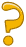x0 = 1，所以。。。 00 = 1 0n = 0，所以。。。 00 = 0 有疑问…… 00 = "不确定"